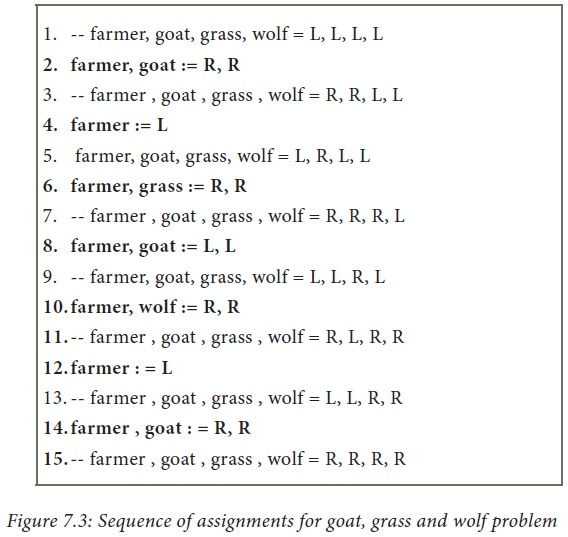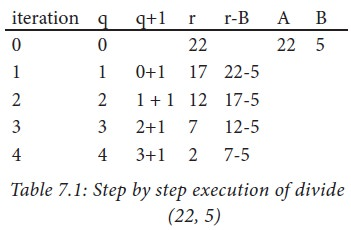Home | | Computer Science 11th std | Composition

# Composition

A statement is a phrase that commands the computer to do an action.

Composition

A statement is a phrase that commands the computer to do an action. We have already seen assignment statement. It is a simple statement, used to change the values of variables. Statements may be composed of other statements, leading to a hierarchical structure of algorithms. Statements composed of other statements are known as compound statements.

Control flow statements are compound statements. They are used to alter the control flow of the process depending on the state of the process. There are three important control flow statements:

•      Sequential

•      Alternative

•      Iterative

When a control flow statement is executed, the state of the process is tested, and depending on the result, a statement is selected for execution.

1. Sequential statement

A sequential statement is composed of a sequence of statements. The statements in the sequence are executed one after another, in the same order as they are written in the algorithm, and the control flow is said to be sequential. Let S1 and S2 be statements. A sequential statement composed of S1 and S2 is written as

o     S1

o     S2

In order to execute the sequential statement, first do S1 and then do S2.

The sequential statement given above can be represented in a flowchart as shown in in Figure 7.2. The arrow from S1 to S2 indicates that S1 is executed, and after that, S2 is executed.Let the input property be P, and the input-output relation be Q, for a problem. If statement S solves the problem, it is written as

-- P

S

-- Q

If we decompose the problem into two components, we need to compose S as a sequence of two statements S1 and S2 such that the input-output relation of S1, say R, is the input property of S2.

-- P

S1

-- R

S2

-- Q

Example 7.1. Let us solve the Farmer, Goat, Grass, and Wolf problem of Example 6.12. We decided to represent the state of the process by four variables farmer, goat, grass, and wolf, representing the sides of the farmer, goat, grass and wolf, respectively. In the initial state, all four variables have the value L (Left side). In the final state, all four variables should have the value R (Right side). The goal is to construct a statement S so as to move from the initial state to the final state.

1.  -- farmer, goat, grass, wolf = L, L,  L,

L

2. S

3. -- farmer , goat , grass , wolf = R, R, R, R

We have to compose S as a sequence of assignment statements such that in none of the intermediate states

1. goat and wolf have the same value but farmer has the opposite value, or

2. goat and grass have the same value but farmer has the opposite value.Subject to these constraints, a sequence of assignments and the state after each assignment are shown in Figure 7.3.Other than lines (1) and (15), in line (7), goat and grass have the same value, but farmer also has the same value as they. In line (9), goat and wolf have the same value, but farmer also has the same value as they. Thus, the sequence has achieved the goal state, without violating the constraints.

2. Alternative statement

A condition is a phrase that describes a test of the state. If C is a condition and both

S1 and S2 are statements, then

if C

S1

else

S2

is a statement, called an alternative statement, that describes the following action:

1.           Test whether C is true or false.

2.           If C is true, then do S1; otherwise do S2.

In pseudo code, the two alternatives S1 and S2 are indicated by indenting them from the keywords if and else, respectively. Alternative control flow is depicted in the flowchart of Figure 2.4. Condition C has two outgoing arrows, labeled true and false. The true arrow points to the S1 box. The false arrow points to the S2 box. Arrows out of S1 and S2 point to the same box, the box after the alternative statement.Conditional statement: Sometimes we need to execute a statement only if a condition is true and do nothing if the condition is false. This is equivalent to the alternative statement in which the else-clause is empty. This variant of alternative statement is called a conditional statement. If C is a condition and S is a statement, then

if C

S

is a statement, called a conditional statement, that describes the following action:

1.           Test whether C is true or false.

2.           If C is true then do S; otherwise do nothing.

The conditional control flow is depicted in the flowchart of Figure 2.5.Example 7.2. Minimum of two numbers: Given two numbers a and b, we want to find the minimum of the two using the alternative statement. Let us store the minimum in a variable named result. Let a ↓ b denote the minimum of a and b (for instance, 4 ↓ 2 = 2, —5 ↓ 6 = -5). Then, the specification of algorithm minimum is

minimum(a, b)

--input s : a , b

--outputs: result = a ↓ b

Algorithm minimum can be defined as

•      minimum(a, b)

•      -- a, b

•      if a < b

•      result : = a

•      else

•      result = b

•      -- result = a ↓ b

3. Case analysis

Alternative statement analyses the problem into two cases. Case analysis statement generalizes it to multiple cases. Case analysis splits the problem into an exhaustive set of disjoint cases. For each case, the problem is solved independently. If C1, C2, and C3 are conditions, and S1, S2, S3 and S4 are statements, a 4-case analysis statement has the form,

case  C1

S1

case  C2

S2

case  C3

S3

else

S4

The conditions C1, C2, and C3 are evaluated in turn. For the first condition that evaluates to true, the corresponding statement is executed, and the case analysis statement ends. If none of the conditions evaluates to true, then the default case S4 is executed.

1.           The cases are exhaustive: at least one of the cases is true. If all conditions are false, the default case is true.

2.           The cases are disjoint: only one of the cases is true. Though it is possible for more than one condition to be true, the case analysis always executes only one case, the first one that is true. If the three conditions are disjoint, then the four cases are (1) C1, (2) C2, (3) C3, (4) (not C1) and (not C2) and (not C3).

Example 7.3. We want an algorithm that compares two numbers and produces the result asWe can split the state into an exhaustive set of 3 disjoint cases: a < b, a = b, and a> b. Then we can define compare() using a case analysis.

1.           compare(a, b)

2.           case a < b

3.           result := -1

4.           case a = b

5.           result := 0

6.           else -- a > b

7.           result : = 1

4. Iterative statement

An iterative process executes the same action repeatedly, subject to a condition C. If C is a condition and S is a statement, then

while C

S

is a statement, called an iterative statement, that describes the following action:

1.           Test whether C is true or false.

2.           If C is true, then do S and go back to step 1; otherwise do nothing.

The iterative statement is commonly known as a loop. These two steps, testing C and executing S, are repeated until C becomes false. When C becomes false, the loop ends, and the control flows to the statement next to the iterative statement. The condition C and the statement S are called the loop condition and the loop body, respectively. Testing the loop condition and executing the loop body once is called an iteration. not C is known as the termination condition.

Iterative control flow is depicted in the flowchart of Figure 7.6. Condition C has two outgoing arrows, true and false. The true arrow points to S box. If C is true, S box is executed and control flows back to C box. The false arrow points to the box after the iterative statement (dotted box). If C is false, the loop ends and the control flows to the next box after the loop.Example 7.4. Construct an iterative algorithm to compute the quotient and remainder after dividing an integer A by another integer B.

We formulated the specification of the algorithm in Example 6.6 as

divide (A , B)

-- inputs: A is an integer and B ≠ 0

--outputs :  q and r such that A = q X B + r and

--0 ≤ r < B

Now we can construct an iterative algorithm that satisfies the specification.

divide (A , B)

--inputs: A is an integer and B ≠ 0

--outputs : q and r such that A = q X B + r and

--0 < r < B

q := 0, A

while r ≥ B

q, r := q + 1, r - B

The algorithm is presented as a flowchart in Figure 7.1.

We can execute the algorithm step-by-step for a test input, say, (A, B) = (22, 5). Each row of Table 7.1 shows one iteration — the evaluation of the expressions and the values of the variables at the end of an iteration. Note that the evaluation of the expression uses the values of the variables from the previous row. Output variables q and r change their values in each iteration. Input variables A and B do not change their values. Iteration 0 shows the values just before the loop starts. At the end of iteration 4, condition (r B) = (2 5) is false, and hence the loop ends with (q, r) = (4, 2).Example 7.5. In the Chameleons of Chromeland problem of Example 1.3, suppose two types of chameleons are equal in number. Construct an algorithm that arranges meetings between these two types so that they change their color to the third type. In the end, all should display the same color.

Let us represent the number of chameleons of each type by variables a, b and c, and their initial values by A, B and C, respectively. Let a = b be the input property. The input-output relation is a = b = 0 and c = A+B+C. Let us name the algorithm monochromatize. The algorithm can be specified as

monochromatize(a, b, c)

--inputs: a=A, b=B, c=C, a=b

--outputs : a = b = 0 , c = A+B+C

In each iterative step, two chameleons of the two types (equal in number) meet and change their colors to the third one. For example, if A, B, C = 4, 4, 6, then the series of meetings will result inIn each meeting, a and b each decreases by 1, and c increases by 2. The solution can be expressed as an iterative algorithm.

monochromatize(a, b, c)

--inputs: a=A, b=B, c=C, a=b

--outputs: a = b = 0, c = A+B+C

while a > 0

a, b, c := a-1, b-1, c+2

The algorithm is depicted in the flowchart of Figure 7.7.Tags : Computer Science , 11th Computer Science : Chapter 7 : Composition and Decomposition
Study Material, Lecturing Notes, Assignment, Reference, Wiki description explanation, brief detail
11th Computer Science : Chapter 7 : Composition and Decomposition : Composition | Computer Science